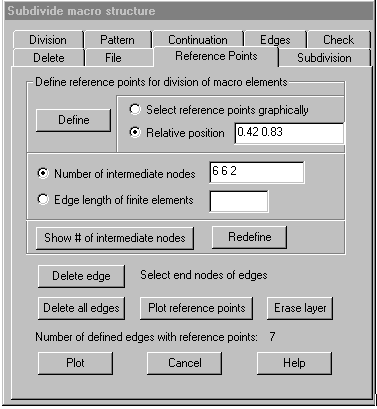Reference Point: Define reference points for division of macro elements

This command allows defining reference points on edges of macro elements that shall become finite element nodes when the macro element is subdivided. The reference points are assigned to edges of macro elements; the corner nodes of these edges must graphically be selected. For each edge with reference points, the number of those points, their relative position and the number of intermediate nodes that should be generated between these points is stored. A maximum of four reference points may be given for one edge. The number of intermediate nodes between the reference points may also be defined using the command Division where each part of edges with reference points is divided individually.

The definition of reference points is saved within the file project.mut.

In most cases following steps may be useful (see demo “referencepoint.dem”).

1) Define the position of reference points, without defining the number of intermediate nodes.

2) Divide all edges using the command Division.

3) Do a graphical test of the subdivision of some elements using command Subdivision and the option “Test individual elements”.

4) If there are triangular finite elements are generated for some macro elements, correct the number of intermediate nodes for some parts of edges with reference points by using the option “Show # of intermediate nodes / Redefine”.

Following dialog shows the available options:Define reference points for division of macro elements

Select reference points graphically: If this option is marked, after selecting the end nodes of an element edge the reference nodes on that edge have to be selected graphically. Selection of reference nodes is finished with the right mouse button, then the next edge may be selected or finish selection with right mouse button. The reference points to be selected must lie within a small tolerance on the edge.

Relative position: Using this option, the relative position (0 < s < 1) for up to 4 reference points must be given in the corresponding input field.

Number of intermediate nodes: Using this option, for each part of the edge the number of intermediate nodes that should be generated when the edge is subdivided must be given in the input field. Missing values are set to 0. The number of intermediate nodes may be omitted when it is later determined by using command Division.

Edge length of finite elements: Using this option, the maximal length of finite element edges must be given in the input field. The parts of the edge are then subdivided as with command Division.

Define: After clicking this button, edges with reference points have to be selected graphically by selecting the end nodes of the edge. Selection is ended with the right mouse button, eventually also the reference nodes on the edges must also be selected graphically if option “select reference points graphically” is marked. If there are already reference points defined for an edge, these are replaced by the new definition.

Show number of intermediate nodes / Redefine: Clicking the first button, an edge must be selected graphically by selecting the end nodes, then the relative position of reference points on this edge and the number of intermediate nodes for the parts of the edge is shown in the corresponding input fields. After that, the number of intermediate nodes may be altered in the input field and be saved for the latest selected edge by clicking the button “Redefine”.

Delete edge

After clicking this button, the end nodes of the edges must be graphically selected that reference points should be deleted. Selection is ended with the right mouse button.

Delete all edges

Clicking this button, all defined reference points are deleted.

Plot reference points

Clicking this button, all edges that have reference points are plotted together with the reference points and the intermediate nodes on the parts of the edge.

Erase layer

Clicking this button, the layers on which the reference points and the intermediate nodes are plotted on are erased.

Plot

Clicking this button, the intermediate nodes on all element edges are plotted.